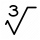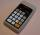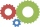# Square root + exponential equation - math problems

#### Number of problems found: 10

• Exponential equationFind x, if 625 ^ x = 5 The equation is exponential because the unknown is in the exponential power of 625
• One third powerWhich equation justifies why ten to the one-third power equals the cube root of ten?
• ExpIf ?, then n is:
• Geometric sequenceIn the geometric sequence is a4 = 20 a9 = -160. Calculate the first member a1 and quotient q.
• What isWhat is the annual percentage increase in the city when the population has tripled in 20 years?
• DecibelBy what percentage does the sound intensity increase if the sound intensity level increases by 1 dB?
• Geometric sequence 3In geometric sequence is a8 = 312500; a11= 39062500; sn=1953124. Calculate the first item a1, quotient q and n - number of members by their sum s_n.
• Six speedsA drilling machine is to have 6 speed ranging from 50 to 750 revolution per minute. If the speed forms a geometric progression, determine their values.
• Annual growthThe population has grown from 25,000 to 33,600 in 10 years. Calculate what was the average annual population growth in%?
• Volume of woodEvery year, at the same time, an increase in the volume of wood in the forest is measured. The increase is regularly p% compared to the previous year. If in 10 years the volume of wood has increased by 10%, what is the number p?

We apologize, but in this category are not a lot of examples.
Do you have an interesting mathematical word problem that you can't solve it? Submit a math problem, and we can try to solve it.

We will send a solution to your e-mail address. Solved examples are also published here. Please enter the e-mail correctly and check whether you don't have a full mailbox.

Please do not submit problems from current active competitions such as Mathematical Olympiad, correspondence seminars etc...

Square root - math word problems. Exponential equation - math word problems.